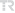## Resolvent Operator of the Matrix Schrödinger Equation on the Half-Line withQuasi-selfadjoint Potential

Gazi University Journal of Science Part A: Engineering and Innovation, vol.8, no.2, pp.197-207, 2021 (Peer-Reviewed Journal)• Publication Type: Article / Article
• Volume: 8 Issue: 2
• Publication Date: 2021
• Journal Name:
• Journal Indexes: TR DİZİN (ULAKBİM), Index Copernicus
• Page Numbers: pp.197-207
• Gazi University Affiliated: Yes

#### Abstract

We obtain the resolvent operator of the matrix Schrödinger equation on the half-line with a quasi-selfadjoint matrix potential Q. We also assume each entry of Q is Lebesgue measurable on (0,∞) and Q has a finite first moment. We impose the general boundary condition at x=0. This boundary value problem is not selfadjoint which makes it valuable and difficult in terms of the spectral analysis. Moreover, considering the most general boundary conditions generalizes many studies in the literature. We introduce the Jost matrix of this boundary value problem. We examine asymptotical and analytical properties of the Jost matrix in order to derive the resolvent operator and point spectrum. We use the quasi-selfadjointness of the matrix potential Q to obtain these properties. We show that the resolvent set consists of squares of the non-singular points of the Jost matrix in the upper complex plane. Moreover, we obtain the Green’s function of this boundary value problem with the help of the Jost matrix. In the light of this main result, we show that the continuous spectrum is [0,∞) and the point spectrum consist of squares of the singular points of the Jost matrix in the upper complex plane. We also show that the set of spectral singularities is empty.